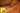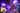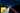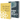# Linear Algebra in JavaScript with Matrix Operations

by Robin WieruchWhen I recently started to dive into the topic of machine learning, I had to relearn all the things I have studied about linear algebra, stochastic and calculus at school and university. I took a little refresher on matrix operations (addition, subtraction, multiplication and division) in linear algebra and learned about the different types of matrices (inverse matrix, transpose matrix, identity matrix) again. The article is a refresher about those things and applies them in JavaScript. Furthermore, in the end of the article, there will be a little example to demonstrate why matrices are beneficial for computations in machine learning. In addition, you will find a couple of tips on how to express mathematical equations in JavaScript similar to Octave or Matlab.

## Linear Algebra in JavaScript

A matrix is just an array in an array when programming with them. In JavaScript, they can be simply expressed as:

`const matrix = [  [0, 1],  [2, 3],  [4, 5],];`

whereas m equals the row and n equals the column of a matrix[m][n]. A vector is a specific kind of a matrix whereas the matrix has only one column.

`const vector = [  ,  ,  ,];`

The most simple mathematical object in linear algebra is a scalar. It is just a single number.

`const scalar = 4;`

Matrices and vectors can be expressed with arrays in programming. But what about matrices with more than two dimensions? They need more than two axes. In general, these arrays of numbers with a variable number of axes are called tensors.

## Matrix Operations in JavaScript

You should be able to apply matrix operations all by yourself, but it can become ugly when using plain JavaScript with loops. Fortunately, there exists a library in JavaScript for mathematics called math.js. Defining a matrix becomes as simple as:

`const matrix = math.matrix([[0, 1], [2, 3], [4, 5]]);`

You can get its dimension by using the `size()` method and its value as array with the `valueOf()` method. Furthermore, you can apply matrix operations such as addition, subtraction, multiplication and division:

`const matrixA = math.matrix([[0, 1], [2, 3], [4, -5]]);const matrixB = math.matrix([[1, -1], [-2, 4], [-7, 4]]); // additionconst matrixAdditionAB = math.add(matrixA, matrixB);// [ [ 1, 0 ], [ 0, 7 ], [ -3, -1 ] ] // subtractionconst matrixAdditionAB = math.subtract(matrixA, matrixB);// [ [ -1, 2 ], [ 4, -1 ], [ 11, -9 ] ] // multiplicationconst matrixK = math.matrix([[0, 1], [2, 3], [4, 5]]);const matrixL = math.matrix([[2, 4], [6, 2]]); const matrixKL = math.multiply(matrixK, matrixL);// [ [ 6, 2 ], [ 22, 14 ], [ 38, 26 ] ] // divisionconst matrixY = math.matrix([[0, 2], [2, 4], [4, 6]]);const matrixZ = math.matrix([[2, 1], [2, 2]]); const matrixYZ = math.divide(matrixY, matrixZ);// [ [ -2, 2 ], [ -2, 3 ], [ -2, 4 ] ]`

Note that for instance the product of a matrix in the case of math.js is not just a new matrix containing the product of the individual matrices. This would be called an element-wise product (or Hardamard product). Instead it is a matrix product operation.

In addition, you can perform matrix scalar multiplication and division as well. It's performed element-wise.

`// matrix scalar multiplicationconst matrixG = math.matrix([[0, 1], [2, 3], [4, -5]]); const matrixG3 = math.multiply(3, matrixG);// [ [ 0, 3 ], [ 6, 9 ], [ 12, -15 ] ] // matrix scalar divisionconst matrixH = math.matrix([[2, 4], [6, 2], [4, -4]]); const matrixH2 = math.divide(matrixH, 2);// [ [ 1, 2 ], [ 3, 1 ], [ 2, -2 ] ]`

Since a vector is only a specific form of a matrix, you can perform matrix-vector multiplication too.

`const matrixI = math.matrix([[0, 1], [2, 3], [4, 5]]);const vectorJ = math.matrix([, ]); const vectorIJ = math.multiply(matrixI, vectorJ);// [ [ 1 ], [ 7 ], [ 13 ] ]`

In case you want to have an element-wise multiplication or division in JavaScript, you can use `math.dotMultiply(matrixI, vectorJ);` or `math.dotDivide(matrixY, matrixZ)`. Otherwise, when using the default operators on matrices with math.js, you will apply the default matrix operations.

After all, dealing with matrices in math.js isn't that difficult anymore. But you have to know the dimensions of each matrix in your operation, because not every matrix operates on another matrix. Another good thing to know are the associative and commutative matrix operations.

## Is matrix multiplication associative and commutative?

There are two important properties for matrix multiplication. First, matrix multiplication is not commutative: A x B != B x A.

`const matrixN = math.matrix([[0, 1], [2, 3]]);const matrixO = math.matrix([[2, 4], [6, 2]]); const matrixNO = math.multiply(matrixN, matrixO);const matrixON = math.multiply(matrixO, matrixN); console.log('Is matrix multiplication commutative?');console.log(math.equal(matrixNO.valueOf(), matrixON.valueOf()));// false`

Second, matrix multiplication is associative: A x (B x C) == (A x B) x C.

`const matrixP = math.matrix([[0, 1], [2, 3], [4, 5]]);const matrixQ = math.matrix([[2, 4], [6, 2]]);const matrixR = math.matrix([[5, 2], [2, -2]]); const matrixPQ_R = math.multiply(math.multiply(matrixP, matrixQ), matrixR);const matrixP_QR = math.multiply(matrixP, math.multiply(matrixQ, matrixR)); console.log('Is matrix multiplication associative?');console.log(math.equal(matrixPQ_R.valueOf(), matrixP_QR.valueOf()));// true`

These matrix multiplication properties should be internalized before making any further more complex operations on matrices.

## Transpose and Inverse and the Identity Matrix in JavaScript

There are a couple of other matrix operations and matrix types in linear algebra. First, the Identity (I) Matrix with the dimension i * j is defined as i-dimensional matrix whereas i == j. The following matrix is an identity matrix.

`const matrix = [  [1, 0, 0],  [0, 1, 0],  [0, 0, 1],];`

In math.js you can use the `eye(i)` method to generate those with the dimension of i.

`const matrixI3 = math.eye(3);// [ [ 1, 0, 0 ], [ 0, 1, 0 ], [ 0, 0, 1 ] ]`

Identity matrices are used later on for more sophisticated matrix operations. Used with another matrix in a matrix operation, identity matrices are a special case because they are commutative: A x I == I x A.

Another type of matrix is the transposed matrix. It is a matrix where the dimensions are flipped. Basically the rows become columns and the columns become rows. In the following example, the vector becomes a so called row vector.

`const matrixV = math.matrix([, , ]); const matrixV_T = math.transpose(matrixV);// [ [ 0, 1, 2 ] ]`

Last but not least, matrices can have an inverse A' but not all matrices (called singular or degenerate) have one. You can find the inverse of a matrix by using the identity matrix: A(A') = (A')A = I.

`const matrixT = math.matrix([[0, 1], [2, 3]]);const matrixU = math.eye(2); const matrixT_I = math.divide(matrixU, matrixT);// [ [ -1.5, 0.5 ], [ 1, -0 ] ]`

Math.js gives you the inverse operation for free. You can use the same matrix from the previous example and call the `inv()` method on it.

`const matrixS = math.matrix([[0, 1], [2, 3]]); const matrixS_I = math.inv(matrixS);// [ [ -1.5, 0.5 ], [ 1, -0 ] ]`

In the end, regardless of the programming language you are using, you will find one powerful math library such as math.js to apply all of these operations.

## How to apply those learnings in Machine learning?

The previous learnings gave a basic understanding of linear algebra with matrices used in JavaScript. How does it help us in machine learning? You can take the example of . Matrix operations can be used to make linear regression simpler to execute and computational efficient. But also other machine learning algorithms in the future. For instance, when having three (trained) competing hypotheses functions for a linear regression, it becomes simple with matrices to calculate their results.

`// Predicting Housing Prices with 3 competing Hypotheses // const HOUSE_SIZES = [2104, 1416, 1534, 852]; // const h1 = x => -40 + 0.25 * x;// const h2 = x => 200 + 0.1 * x;// const h3 = x => -150 + 0.4 * x; const houseSizeMatrix = math.matrix([  [1, 2104],  [1, 1416],  [1, 1534],  [1, 852],]); const hypothesesMatrix = math.matrix([  [-40, 200, -150],  [0.25, 0.1, 0.4],]); const competingResults = math.multiply(houseSizeMatrix, hypothesesMatrix); // Column: Result for each Hypothesis// Row: Result for each House Size // [//  [ 486, 410.4, 691.6 ],//  [ 314, 341.6, 416.4 ],//  [ 343.5, 353.4, 463.6 ],//  [ 173, 285.2, 190.8 ],// ]`

You can put these computations in matrices now, rather than executing every function on its own. A loop becomes one matrix operation. On a higher level you can say that a . Thus it becomes computational efficient when performing machine learning algorithms and simpler as well. Furthermore, these matrix operations are used in a by default which is used as an alternative to gradient descent.

## Octave / Matlab alike operations in JavaScript

At some point, using math.js the proposed way doesn't scale anymore. You will do more than one matrix operation in complex mathematical expressions. What about the following expressions?

`theta - ALPHA / m * ((X * theta - y)' * X)'`

Yes, it is taken from a . It can be easily expressed in mathematical programming languages such as Matlab or Octave. In math.js it wouldn't scale when using the standard methods.

`// Octave:// theta = theta - ALPHA / m * ((X * theta - y)' * X)'; // Math.js in JavaScripttheta = math.subtract(  theta,  math.multiply(    (ALPHA / m),    math.transpose(      math.multiply(        math.transpose(          math.subtract(            math.multiply(              X,              theta            ),            y          )        ),        X      )    )  ));`

That's a mess. However, fortunately you can do it concise by using the eval functionality that takes a mathematical expression and the scoped values to apply those in the expression.

`// Octave:// theta = theta - ALPHA / m * ((X * theta - y)' * X)'; // Math.js in JavaScripttheta = math.eval(`theta - ALPHA / m * ((X * theta - y)' * X)'`, {  theta,  ALPHA,  m,  X,  y,});`

Still not as concise as using Octave or Matlab, but you can evaluate complex mathematical expression now. It helps you in other scenarios too. For instance, it can be used to extract a subset of a Matrix by range indices:

`// Octave:// matrixAsub = matrixA(:, 1:2); // Math.js in JavaScriptlet matrixAsub = math.eval('matrixA[:, 1:2]', {  matrixA,});`

It returns the first and second column (indices start with 1) with all their rows as two vectors in a new matrix. It goes even further by assigning columns in a matrix a new vector.

`// Octave:// matrixA(:, 1) = vectorB; // Math.js in JavaScriptmath.eval(`matrixA[:, 1] = vectorB`, {  matrixA,  vectorB,});`

In conclusion, I hope the walkthrough about matrices applied in JavaScript was helpful to get started in the linear algebra in JavaScript or as foundation for machine learning in JavaScript. You can checkout the GitHub repository with executable matrix operations on the command line. If you like it, make sure to star it.## Neural Networks in JavaScript with deeplearn.js

A couple of my recent articles gave an introduction into a subfield of artificial intelligence by implementing foundational machine learning algorithms in JavaScript (e.g. linear regression with…## Polynomial Regression and Model Selection

After learning about gradient descent in a linear regression , I was curious about using different kinds of hypothesis functions to improve the result of the algorithm itself. So far, the hypothesis…